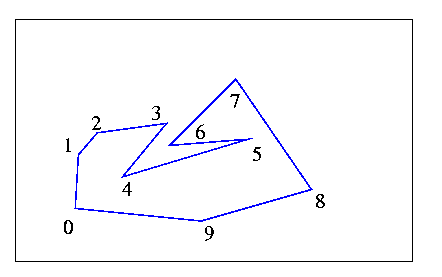Orlowski 1985

Orlowski states that his algorithm  is "based on a slight improvement of Sklansky's (original) algorithm". His algorithm has the same basic idea as those of Lee, Bhattacharya and ElGindy, Preparata and Shamos. In his introduction he only refers to Lee's algorithm.

Assume that the polygon is ordered clockwise, with the first vertex at Xmax. The convex hull vertices are to be stored in a stack. The top three vertices of the stack will always be i, j and k (k being the top). Orlowski uses a "dummy" point with index zero. X0 = X1 and Y0 < X0, so that 0,1,2 form a convex turn. The algorithm is shown below:

(0) Initialize the stack to contain [0,1,2,3].

(1) If k = m+2, DONE,

else go to (2).

(2) If (i,j,k) don't form a left turn, go to (3)

else delete j and go to (2).

(3) If (j-1,j,k) don't form a left turn, push k+1 and go to (1).

else push k+1, delete j, and go to (4).

(4) If (i,j,k) don't form a left turn, push k+1, delete j, and go to (4).

else delete j and go to (2).

Note that j is always the second element from the top of the stack. So if k+1 is pushed (ex: in 4), j changes.

This algorithm adds one pocket test to Sklansky's original algorithm. Recall that Sklansky's algorithm would fail for the polygon below. Vertices (3,4,5) would form a left turn, so 4 would be deleted. The algorithm would backtrack to check vertices (2,3,5) and from then on, every triplet of vertices forms a convex turn. Orlowski's algorithm would delete vertex 4, but then it would delete all vertices that are inside the pocket which has edge (3,5) as its lid. Lee's algorithm (and others) do the same thing. This algorithm just does not include any other pocket tests. Although this means that fewer cases are checked in every iteration, it is not necessary that the algorithm is "better". Please see the end of the section on Shin and Woo for similar comments. Essentially, this algorithm is identical to that of Shin and Woo.There are a couple of "clerical" errors in the article, on p.362: in step 4b, the "<" should be replaced with a ">". Also in one of the boxes in the diagram, "i:=B(i)" should follow "j:=1", otherwise j is set equal to i.

previous : Preparata & Shamos 1985.          next : Shin & Woo 1986.